# 针对交易的组合数学和概率论（第三部分）：第一个数学模型

14 十月 2021, 14:15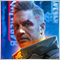0
466

### 新思路

1. S = K^2 - 新走廊的平均步阶数，基于步阶是另一条走廊的事实
2. P = K * P0  --> K = P/P0 - 已知走廊比未知走廊大多少倍
3. P - 平均步阶数未知的走廊宽度（步阶数是原走廊大小的一半）
4. P0 - 已知走廊的宽度

• S = n * m - 走廊有一半不对称的平均步阶数
• n - 适合走廊上半部分的步阶数
• m - 适合走廊下半部分的步阶数

• S = n * n = m * m = m * n = n^2 = m^2 = K^2
• K = n = m

• S(n*k ,m*b) = m*k*n*b = n*m * k*b
• S(n ,m) * S(k ,b) = n*m * k*b

Before proceeding with the fractals, let me remind you that the above defined formulas only work if p=0.5. As soon as the market or fractal parameters begin to deviate from a random walk, this formula begins to change very interestingly, namely:

• Ss[n,m,p] – a more general formula for the average number of steps (in any direction)
• S(n ,m)  = Ss[n,m,0,5] – formula for a random walk is a special case of the general formula

### 平均步阶数的一般公式

1.  Ss[n,m,1] = Sn[n] = n
2. Ss[n,m,0] = Sm[m] = m
3.  Ss[n,m,0.5] = Sn[n] * Sm[m] = m*n
4.  Sn[n,p] – 单一方向到上边界的步阶数
5. Sn[m,p] – 单一方向到下边界的步阶数

• A^X * B^X = (A*B)^X

• Ss[m,n,p] = ( Sn[n] ^ Pn[p] ) * ( S[m] ^ Pm[p] ) = (n ^ Pn[p] ) * ( m ^ Pm[p] )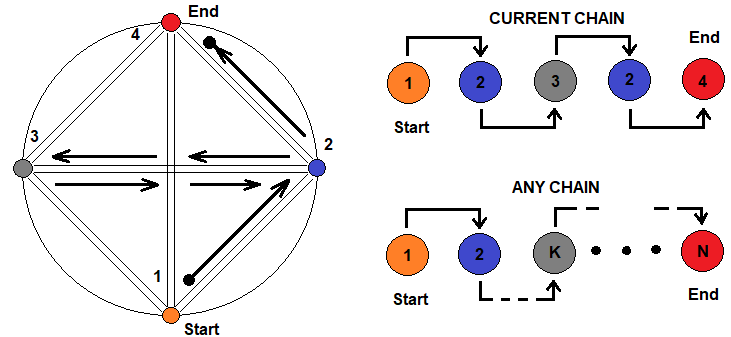### 构建幂函数的原型

• Pn[p] – 上边界乘数的幂
• Pm[p] – 下边界乘数的幂

• Pn[0.5] = 1 , Pn = 1 , Pn = 0
• Pm[0.5] = 1 , Pm = 1 , Pm = 0

1. Pn[p] = 1 , if p >= 0.5
2. Pn[p] = ( (1 – p)/0.5 ) ^ K
3. Pm[p] = 1 , if p <= 0.5
4. Pm[p] = ( p/0.5 ) ^ K
5. K 是调节函数平坦度的幂

• Pn[p] = C1 * ( (1 – p)/0.5 ) ^ K1 + C2 * ( (1 – p)/0.5 ) ^ K2 … + … + CN * ( (1 – p)/0.5 ) *KN
• Pm[p] = C1 * ( p/0.5 ) ^ K1 + C2 * ( p/0.5 ) ^ K2 … + … + CN * ( p/0.5 ) ^ KN
• С1 + С2 + … + … СN = 1是相关幂的权重
• KN 是对应项的幂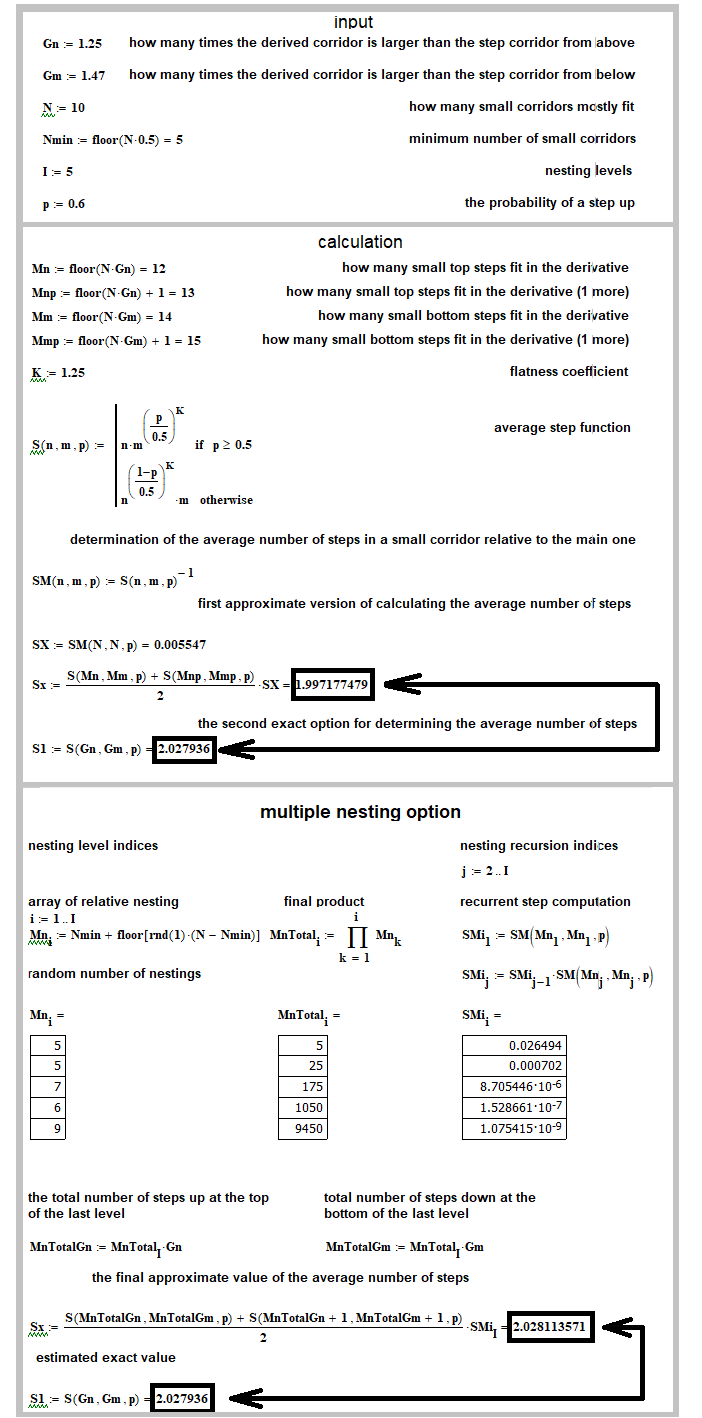### 从原型受限族接收最佳函数的一般规划案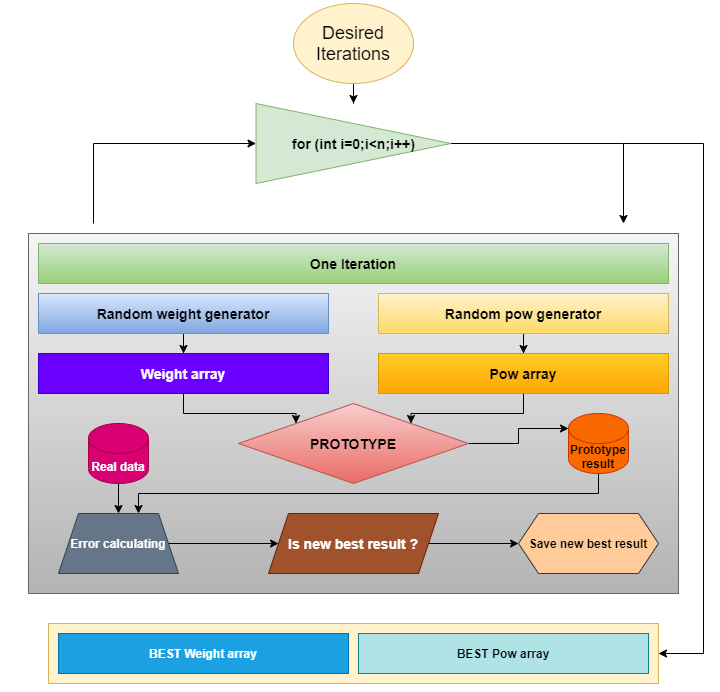### 深入分析

• SP[U,ud] = S[U,u] * P[U] + S[U,d] * P[U] = (S[U,u] + S[U,d]) * P[U] = S[U] * P[U]
• S[U] – 如果步阶链首先到达上边界，则为步阶的平均数
• S[U,u] – 如果步阶链首先到达上边界，则为平均向上步阶数
• S[U,d] – 假设步阶链首先到达上边界的平均步阶数
• P[U] – 价格首先跨越上边界的概率
• SP[U,ud] – 跨越上边界的平均可能步阶数

• SP[D,ud] = S[D,u] * P[D] + S[D,d] * P[D] = (S[D,u] + S[D,d]) * P[D] = S[D] * P[D]
• S[D] – 如果步阶链首先到达下边界，则为任何步阶的平均数
• S[D,u] – 如果步阶链首先到达下边界，则为平均向上的步阶数
• S[D,d] – 假设步阶链首先到达下边界的平均步阶数
• P[D] – 价格首先跨越下边界的概率
• SP[D,ud] – 跨越下边界的平均可能步阶数

• S = SP[U,ud] + SP[D,ud]

• S[U,u] = SP[U,u]/P[U] – 如果步阶链首先到达上边界，则为平均向上步阶数
• S[U,d] = SP[U,d]/P[U] – 假设步阶链首先到达上边界的平均步阶数
• S[D,u] = SP[D,u]/P[D] – 如果步阶链首先到达下边界，则为平均向上的步阶数
• S[D,d] = SP[D,d]/P[D] – 假设步阶链首先到达下边界的平均步阶数
• P[U] – 价格首先跨越上边界的概率

• SP[U,u] – 如果步阶链首先到达上边界，则平均向上步阶数
• SP[U,d] – 假设步阶链首先到达上边界的平均步阶数
• SP[D,u] – 如果步阶链首先到达下边界，则平均向上的步阶数
• SP[D,d] – 假设步阶链首先到达下边界的平均步阶数
• P[U] – 价格首先跨越上边界的概率
• P[D] – 价格首先跨越下边界的概率
• S = SP – 任何步阶的平均数

• SP[U]
• S[U,ud] = S[U,u] + S[U,d]
• S[D,ud] = S[D,u] + S[D,d]
• P[D] = 1 – P[U]
• S = SP[U,ud] + SP[D,ud]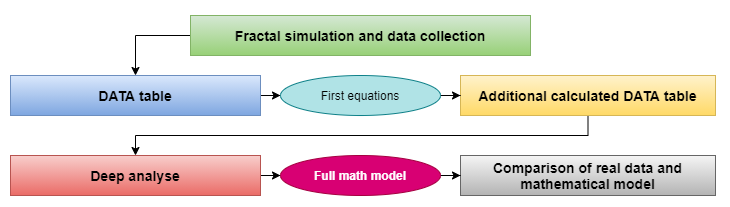### 数据集合和结论

 测试索引 走廊上半部分的步阶 (n) 走廊下半部分的步阶 (n) ( m ) 初始步阶的概率   ( p ) 上边界的平均可能向上步阶数 ( SP[U,u]  ) 上边界的平均可能向下步阶数 ( SP[U,d]  ) 下边界的平均可能向上步阶数 ( SP[D,u]  ) 下边界的平均可能向下步阶数 ( SP[D,d]  ) 上边界的任何步阶的平均可能数 ( SP[U,ud]  ) 下边界的任何步阶的平均可能数 ( SP[D,ud]  ) 1 1 1 0.5 0.5 0 0.0 0.5 0.5 0.5 2 2 2 0.5 1.5 0.5 0.5 1.5 2.0 2.0 3 3 3 0.5 3.0 1.5 3.0 1.5 4.5 4.5 4 1 2 0.5 0.888888 0.2222222 0.111111 0.777777 1.11111111 0.8888888 5 2 3 0.5 2.2 1.0 0.8 2.0 3.2 2.8 6 1 2 0.6 1.038781 0.249307 0.066481 0.487534 1.288088 0.554016 7 2 3 0.6 2.811405 1.191072 0.338217 0.906713 4.0024777 1.244931 8 2 3 1.0 2.0 0.0 0.0 0.0 2.0 0.0 9 1 3 0.5 1.25 0.5 0.25 1 1.75 1.25 10 1 4 0.5 1.6 0.8 0.4 1.2 2.4 1.6

 测试索引 走廊上半部分的步阶 (n) 走廊下半部分的步阶 (n) ( m ) 跨越上边界概率 ( P(U) ) 跨越下边界概率 ( P(D) ) 跨越上边界时任何步阶的平均数 (S[U,ud]) = SP[U,ud]/P[U] 跨越下边界时任何步阶的平均数 (S[D,ud]) = SP[D,ud]/P[D] 上边界的平均上升步阶数 ( S[U,u]  ) = SP[U,u]/P[U] 上边界的平均上降步阶数 ( S[U,d]  ) = SP[U,d]/P[U] 下边界的平均上升步阶数 ( S[D,u]  ) = SP[D,u]/(P[D]) 下边界的平均 下降步阶数 ( S[D,d]  ) = SP[D,d]/(P[D]) 平均步阶数   ( S ) 1 1 1 0.5 0.5 1.0 1.0 1.0 0.0 0.0 1.0 1 2 2 2 0.5 0.5 4.0 4.0 3.0 1 1 3 4 3 3 3 0.5 0.5 9.0 9.0 6 3 3 6 9 4 1 2 0.66666 0.3333333 1.6666666 2.6666666 1.3333333 0.33333333 0.33333333 2.33333333 2 5 2 3 0.6 0.4 5.3333333 7 3.6666666 1.66666666 2 5 6 6 1 2 0.789473 0.210526 1.631579 2.631579 1.315790 0.315789 0.315789 2.315789 1.842104 7 2 3 0.810166 0.189498 4.940318 6.569626 3.470159 1.470157 1.784805 4.784 5.2474087 8 2 3 1.0 0.0 2.0 0.0 2.0 0.0 0.0 0.0 2.0 9 1 3 0.75 0.25 2.3333333 5 1.6666666 0.6666666 1 4 3 10 1 4 0.8 0.2 3.0 8.0 2 1 2 6 4

• S[U,u] – S[U,d] = n
• S[D,d] – S[D,u] = m

1. Lim[N0 -- > +infinity] (N0[U]/N0) = p
2. Lim[N0 -- > +infinity] (N0[D]/N0) = 1-p
3. Lim[N0 -- > +infinity] (N0[U]/N) = S[UD,u]
4. Lim[N0 -- > +infinity] (N0[D]/N) = S[UD,d]
5. Lim[N0 -- > +infinity] (N0/N) = S
6. N0 – 与初始步阶相关的基础实验的数量（组成一个复杂的实验）
7. N – 由简单实验组成的复杂实验的数量

• Lim[N0 -- > +infinity] (N0[U]/N) = Lim[N0 -- > +infinity] (N0[U]/N0 * N0/N) = Lim[N0 -- > +infinity] (N0[U]/N0)  * Lim[N0 -- > +infinity] (N0/N) =  p*S  = S[UD,u]
• Lim[N0 -- > +infinity] (N0[D]/N) = Lim[N0 -- > +infinity] (N0[D]/N0 * N0/N) = Lim[N0 -- > +infinity] (N0[D]/N0)  * Lim[N0 -- > +infinity] (N0/N) = (p-1)*S  = S[UD,d]

• S[UD,u] = S*p
• S[UD,d] = S*(1-p)

• S[U,u] * P[U] + S[D,u] * ( 1 – P[U] ) = S*p
• S[U,d] * P[U] + S[D,d] * ( 1 – P[U] ) = S*(1-p)

• Lim[Nt[U] -- > +infinity] ((N0[U] - N0[D])/(Nt[U] - Nt[D]) = 1
• Nt[U] =  – 基准步阶的理想数量，利用基准向上步阶的概率计算
• Nt[D] – 理想的基准步阶数，利用基准向下步阶的概率计算
• N0[U] – 向上的实际基准步阶数
• N0[D] – 向下的实际基准步阶数

• Lim[Nb[U] -- > +infinity] ((N0[U] - N0[D])/(Nb[U] - Nb[D]) = 1
• Nb[U] – 基准步阶的理想数量，利用跨越上边界的概率计算
• Nb[D] – 基准步阶的理想数量，利用跨越下边界的概率计算
• N0[U] – 向上的实际基准步阶数
• N0[D] – 向下的实际基准步阶数

• P[U] * n – (1 - P[U]) * m = p * S - (1 - p) *S

1. S[U,u] – S[U,d] = n
2. S[D,d] – S[D,u] = m
3. S[U,u] * P[U] + S[D,u] * ( 1 – P[U] ) = S*p
4. S[U,d] * P[U] + S[D,d] * ( 1 – P[U] ) = S*(1-p)
5. P[U] * n – (1 - P[U]) * m = p * S - (1 - p) *S = 2*p*S - S

• -->  S[U,u] = n + S[U,d] – 排除 “S[U,u]”
• -->  S[D,d] = m + S[D,u] – 排除 “S[D,d]”
• (n + S[U,d]) * P[U] + S[D,u] * ( 1 – P[U] ) = S*p – 将所有内容替换为等式 3
• S[U,d] * P[U] + (m + S[D,u]) * ( 1 – P[U] ) = S*(1-p) – 将所有内容替换为等式 4

### 计算整个数学模型的算法

• S[U,d] = S[U,u] – n

• S[D,u] = ( S*p – S[U,u] * P[U] ) / ( 1 – P[U] )

• S[D,d] = ( S*(1-p) - S[U,d] * P[U]) / ( 1 – P[U] ) =  ( S*(1-p) - ( S[U,u] – n ) * P[U] ) / ( 1 – P[U] )

• P[U] * n – (1 - P[U]) * m = 2*p*S – S
• --> P[U] * (n + m)  =   2 * p * S – S + m
• --> P[U] = ( 2 * p * S – S + m ) / (n + m)

• n – 到上边界的步阶数
• m – 到下边界的步阶数
• p – 初始向上步阶的概率
• S[U,u] – 如果跨越上边界，则平均上升步阶数
• P[U] – 跨越上边界的概率

• S[U,u] = Ss[m,n,p] = (n ^ Pn[p] ) * ( m ^ Pm[p] )
• Pn[p] = 1 , if p >= 0.5
• Pn[p] = ( (1 – p)/0.5 ) ^ K
• Pm[p] = 1 , if p <= 0.5
• Pm[p] = ( p/0.5 ) ^ K
• K 是调节函数平坦度的幂

1. S[U,u] = Summ[ i = 0, n] ( i ) ; if n == m
2. S[U,d] = Summ[ i = 0, n] ( i-1 ) ; if n == m

• S[D,u] = S[D,d] – m

• S[U,d] = ( S*(1-p) - S[D,d] * ( 1 – P[U] ) ) / P[U]

• S[U,u] = ( S*p – ( S[D,d] – m ) * ( 1 – P[U] ) ) / P[U]

1. m > n, p = 0,5
2. S[U,u] = Summ[ i = 0 … n] ( i ) + (m-1)/3

1. m < n, p = 0,5
2. S[D,d] = Summ[ i = 0 … m] ( i ) + (n-1)/3

1. m = n, p = 0,5
2. S[U,u] = Summ[ i = 0 … n] ( i )
3. S[D,d] = Summ[ i = 0 … m] ( i )

### 获得终极方程的原型

1. Sp[U,u] = S[U,u] ^ K(p)
2. Sp[D,d] = S[D,d] ^ K(q)
3. = 1-p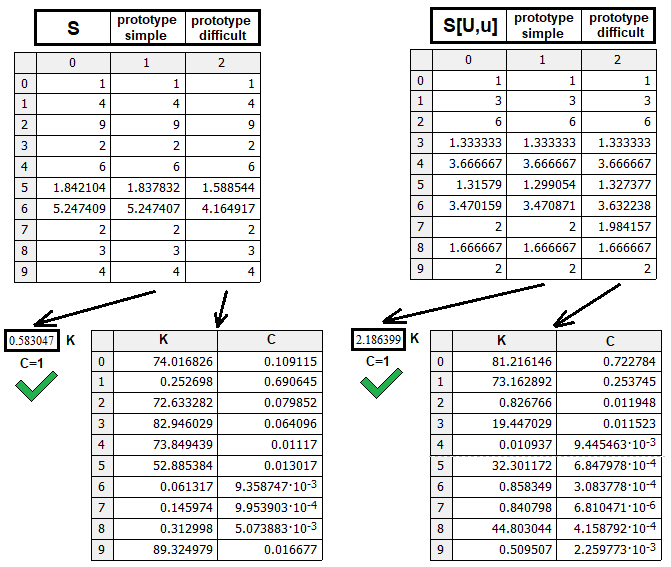1. p = 0.5 ; Sp[U,u] = S[U,u] --> K(0.5) = 1 ,
2. p = 1.0 ; Sp[U,u] = n = S[U,u]^(Log[S[U,u]-->n]) --> K(1.0) = Log[S[U,u]-->n]
3. p = 0.0 ; Sp[U,u] = 0 = S[U,u]^(-infinity) --> K(0.0) = -infinity
4. q = 0.5 ; Sp[D,d] = S[D,d] --> K(0.5) = 1 ,
5. q = 1.0 ; Sp[D,d]  = n = S[D,d]^(Log[S[U,u]-->m]) --> K(1.0) = Log[S[D,d]-->m]
6. q = 0.0 ; Sp[D,d] = 0 = S[D,d]^(-infinity) --> K(0.0) = -infinity

• K(p) = 1 + D * Summ(N) (Kn *  | (( p – 0.5 )/(0.5*p)) | ^ A  + Kn * | (( p – 0.5 )/(0.5*p)) | ^ A + …. + Kn[N] * | (( p – 0.5 )/(0.5*p)) | ^ A[N])
• Kn + Kn + …. Kn[N] = Log[S[U,u]-->n] – 1
• D = (( p – 0.5 )/(0.5*p)) / | (( p – 0.5 )/(0.5*p)) |
• K(q) = 1 + C * Summ(N) (Km * (( q – 0.5 )/(0.5*q)) ^ B  + Km * (( q – 0.5 )/(0.5*q)) ^ B + …. + Km[N] * (( q – 0.5 )/(0.5*q)) ^ B[N])
• Km + Km + …. Km[N] = Log[S[D,d]-->m] – 1
• C = (( q – 0.5 )/(0.5*q)) / | (( q – 0.5 )/(0.5*q)) |
• Kn, Km – 相应项的权重
• A, B - 项式的敏感度

### 数学模型实现与测试

```struct MathModel1//structure for the first mathematical model
{
double S;//the average number of any steps
double pU;//the probability that the price will first reach the upper border
double pD;//the probability that the price will first reach the lower border
double SUu;//the average number of steps up if the price first reaches the upper border
double SUd;//the average number of steps down if the price first reaches the upper border
double SDu;//the average number of steps up if the price first reaches the lower border
double SDd;//the average number of steps down if the price first reaches the lower border

double SPUu;//the average probable number of steps up if the price first reaches the upper border
double SPUd;//the average probable number of steps down if the price first reaches the upper border
double SPDu;//the average probable number of steps up if the price first reaches the lower border
double SPDd;//the average probable number of steps down if the price first reaches the lower border

double SPUud;//the average probable number of steps in any direction if the price first reaches the upper border
double SPDud;//the average probable number of steps in any direction if the price first reaches the lower border

double SUDu;//the average number of steps up when reaching any of the borders
double SUDd;//the average number of steps down when reaching any of the borders
};

double Ss(int n, int m,double p, double K)//prototype of the function of the average number of steps in any direction when reaching any border
{
if (p>=0.5) return n*MathPow(m,MathPow((1-p)/0.5,K));
else return MathPow(n,MathPow(p/0.5,K))*m;
}

double Log(double a, double b)//logarithm function for any base
{
if (MathLog(a) != 0) return MathLog(b)/MathLog(a);
else return 0.0;
}

double SUu(int n,int m)//average number of steps up to the upper border if p=0.5
{
double rez=0.0;
if (m>n)
{
for (int i=0;i<=n;i++) rez+=double(i);
rez+=(m-1)/3.0;
}
if (m==n) for (int i=0;i<=n;i++) rez+=double(i);
return rez;
}

double SDd(int n,int m)//average number of steps down to the lower border if p=0.5
{
double rez=0.0;
if (n>m)
{
for (int i=0;i<=m;i++) rez+=double(i);
rez+=(n-1)/3.0;
}
if (m==n) for (int i=0;i<=m;i++) rez+=double(i);
return rez;
}

double KpnEasy(int n,int m, double p,double A)//power prototype for steps up m>=n
{
double D;
if ( p-0.5 != 0 ) D=(p-0.5)/MathAbs(p-0.5);
else D=1.0;
return 1.0 + D*(Log(SUu(n,m),n) - 1)*MathPow(((p-0.5)/(0.5*p)),A);
}

double KpmEasy(int n,int m,double p,double A)//power prototype for steps down m<n
{
double D;
if ( 0.5-p != 0 ) D=(0.5-p)/MathAbs(0.5-p);
else D=1.0;
return 1.0 + D*(Log(SDd(n,m),m) - 1)*MathPow(((0.5-p)/(0.5*(1.0-p))),A);
}

double SUuS(int n,int m,double p, double A)//full prototype for average steps up m>=n
{
return MathPow(SUu(n,m),KpnEasy(n,m,p,A));
}

double SDdS(int n,int m,double p, double A)//full prototype for average steps down  n>m
{
return MathPow(SDd(n,m),KpmEasy(n,m,p,A));
}

MathModel1 CalculateMathModel(int n, int m, double p,double K=0.582897,double A=2.189246)//calculating the entire mathematical model
{
MathModel1 Mt;
if ( m >= n )
{
Mt.S=Ss(n,m,p,K);
Mt.pU=(2*p*Mt.S-Mt.S+m)/(n+m);
Mt.pD=1.0-Mt.pU;
Mt.SUu=SUuS(n,m,p,A);
Mt.SUd=Mt.SUu-n;
if (1.0-Mt.pU != 0.0) Mt.SDu=(Mt.S*p-Mt.SUu*Mt.pU)/(1.0-Mt.pU);
else Mt.SDu=0.0;
if (1.0-Mt.pU != 0.0) Mt.SDd=(Mt.S*(1.0-p)-Mt.SUd*Mt.pU)/(1.0-Mt.pU);
else Mt.SDd=0.0;
}
else
{
Mt.S=Ss(n,m,p,K);
Mt.pU=(2*p*Mt.S-Mt.S+m)/(n+m);
Mt.pD=1.0-Mt.pU;
Mt.SDd=SDdS(n,m,p,A);
Mt.SDu=Mt.SDd-m;
if (Mt.pU != 0.0) Mt.SUd=(Mt.S*(1.0-p)-Mt.SDd*(1.0-Mt.pU))/Mt.pU;
else Mt.SUd=0.0;
if (Mt.pU != 0.0) Mt.SUu=(Mt.S*p-Mt.SDu*(1.0-Mt.pU))/Mt.pU;
else Mt.SUu=0.0;
}

Mt.SPUu=Mt.SUu*Mt.pU;
Mt.SPUd=Mt.SUd*Mt.pU;
Mt.SPDu=Mt.SDu*Mt.pD;
Mt.SPDd=Mt.SDd*Mt.pD;

Mt.SPUud=Mt.SPUu+Mt.SPUd;
Mt.SPDud=Mt.SPDu+Mt.SPDd;

Mt.SUDu=Mt.SPUu+Mt.SPDu;
Mt.SUDd=Mt.SPUd+Mt.SPDd;

return Mt;
}
```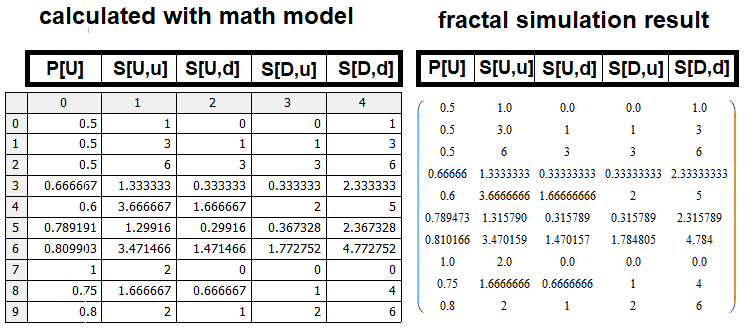### 参考

• #### 针对交易的组合数学和概率论（第一部分）：基础知识

Materials.zip (312.85 KB)

#### 该作者的其他文章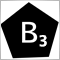更好的程序员（第 03 部分）：放弃做这 5 件事成为一名成功的 MQL5 程序员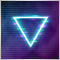针对交易的组合数学和概率论（第二部分）：通用分形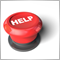MQL5.community - 用户手册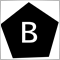更好的程序员（第 02 部分）：停止做这 5 件事变为一名成功的 MQL5 程序员